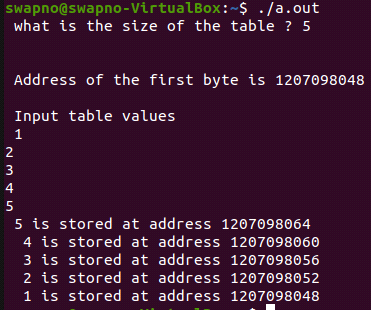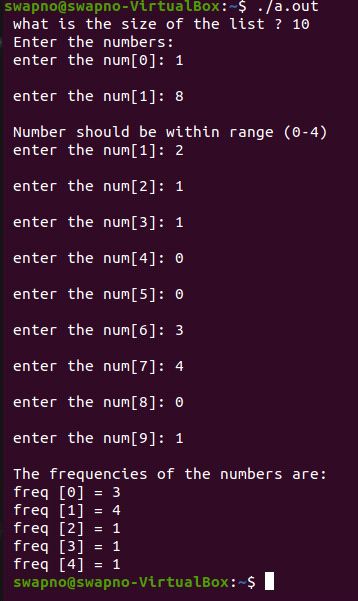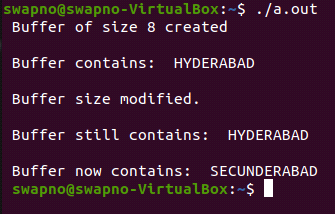| by Arround The Web | No comments

## Dynamic Memory Allocation in C

In DMA, the decision on memories that are allocated cannot take during the compile time. This decision or memory is allocated during the Runtime.

Whenever we create any variable through DMA, that type of variables do not have any name; we access these variables through address or pointer.

In SMA, Programmer knows from earlier time that how many Variables or How many memories are required for his/her program.

But In DMA,  programmer does not know from earlier state that how many variables or memory is required, it depends upon the user’s requirement.

## malloc ()

malloc () function is an action statement when compiler read this line. Compiler doesn’t understand how many memories is allocated as it is an action statement. In runtime memory block is created.

Whenever we call malloc () we pass a number as an argument, which it can understand the number of bytes of memory block are to be created by the malloc (). In malloc (), it can’t declare any data type. Malloc () always return the address, which memory block is created.

Malloc () return type is a void pointer because it doesn’t know which types of address it returns. For this we have to type caste.

 1 P = (float*) malloc (4);

Here we type caste, because malloc () is a void pointer.

## Example-1:

 12345678910111213141516171819202122232425262728 #include #include #define NULL 0 int main () {     int *a , *t ;     int size ;     printf ( " what is the size of the table ? " ) ;     scanf("%d",&size);     printf ( " \n " ) ;     if ( ( t = ( int* ) malloc ( size * sizeof ( int ) ) )  == NULL )     {     printf( " No space available \n " ) ;     exit ( 1 ) ;     }     printf ( " \n Address of the first byte is %u\n " , t ) ;     /* Reading table values*/     printf ( " \n Input table values \n " ) ;     for ( a = t ; a < t + size ; a++ )     scanf("%d", a);     /* Printing table values in reverse order*/     for ( a = t + size - 1 ; a >= t ; a -- )     printf ( " %d is stored at address %u \n ", *a , a ) ;     free ( t ) ;     return 0 ;

## Output:## Calloc ():

With the help of calloc () we can create more than one block or array in calloc (we pass two arguments; 1st one is how many blocks we want to create & 2nd one is the size of the block). calloc () also return address in each block by default 0 is exist.

## Example-2:

 1234567891011121314151617181920212223242526272829303132 #include #include int main () {     int *n , *freq , i , size ;     printf ( " what is the size of the list ? " ) ;     scanf("%d",&size);     n = ( int* ) malloc ( size * sizeof( int ) ) ;     printf ( " Enter the numbers: " ) ;     for ( i = 0 ; i < size ; i++ )     {         printf ( " \n enter the num[%d]: ",i ) ;         scanf("%d",&n[i]);         if ( n [ i ] < 0 || n [ i ] > 4 )             {                 printf ( " \n Number should be within range (0-4) " ) ;                 i-- ;                 continue ;             }     }     freq = ( int * ) calloc ( 5 , sizeof ( int ) ) ;     for ( i = 0 ; i < size ; i++ )             freq [ n [ i ] ]++ ;     printf ( " \n The frequencies of the numbers are: " ) ;     for ( i = 0 ; i < 5 ; i++ )             printf ( " \n freq [%d] = %d " , i , freq [ i ] ) ;     printf ( " \n " ) ;     free ( freq ) ;     return 0 ; }

## Output:## realloc ()

Whenever we create a block with the help of malloc () or calloc () & we want to change or resize the block, we use realloc ().

 1 Void *realloc (void *block, int size)

In realloc() we have to pass the address as an argument from which block we want to resize.

 1 realloc (ptr,8);

and the size of the block we want to resize. That size we have to pass an argument in realloc ().

 123 double *q; q=realloc (ptr,8);

Only those blocks which are created by malloc () or calloc () can be resized by realloc ().

## Example-3:

 1234567891011121314151617181920212223242526272829303132333435363738394041424344454647484950515253545556575859 #include #include #include #define NULL 0 int main() { char *buffer ; /* Allocating memory */ if ( ( buffer = ( char * ) malloc ( 10 ) ) == NULL ) { printf (" malloc failed. \n " ) ; exit ( 1 ) ; } printf ( " Buffer of size %d created \n " , sizeof (buffer) ); strcpy ( buffer , " HYDERABAD " ) ; printf( " \n Buffer contains: %s \n " , buffer ) ; /* Reallocation */ if ( ( buffer = ( char * ) realloc ( buffer , 15 ) ) == NULL ) { printf ( " Reallocation failed. \n " ) ; exit ( 1 ) ; } printf ( " \n Buffer size modified. \n " ) ; printf ( " \n Buffer still contains: %s \n " , buffer ) ; strcpy ( buffer , " SECUNDERABAD " ) ; printf ( " \n Buffer now contains: %s \n " , buffer ) ; /* Freeing memory */ free ( buffer ) ; return 0 ; }

## Output:## free ()

With the help of free (), we release the memory block that is created by malloc () or calloc () or realloc ().

Static variables only exist into the scope of the block or a function. If we cannot run the free (), whenever static variable p is destroyed, the variable which is created dynamically, that are not destroyed, but stayed forever in RAM or in memory. This is called memory leak. For this free () is required to destroy the memory block that is created dynamically.

Free () only destroy those memory that is created dynamically.

## Conclusion:

DMA is a powerful concept in C language because it removes the drawback of SMA. In SMA we have to make decision before running the program that how many memory blocks are created. As a result, memory is wasted or memory is not enough. DMA resolve the problem by taking decision on run time that how many block are required to allocate memory. It allocates memory to the requirement of the program.

Source: linuxhint.com